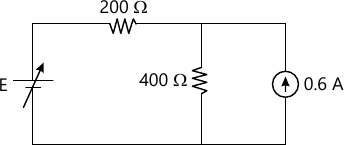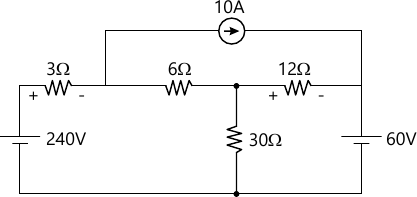# BEE Basic Electricity Electronics question paper May 2012

BEE Basic Electricity Electronics question paper May 2012 – The First year F.E question paper for Mumbai University students of semester 1 B.E.E is as follows:

### BEE Basic Electricity Electronics question paper May 2012

Write Any Four
1 (a) To what voltage should adjustable source E be set in order to produce a current of 0.3 A in 400 ohms resistor.5 M
1 (b) A series RLC circuit is connected to the ac voltage v(t)=24Sin(1000t + 20′) V. if R=15 ohms and L=0.1 H, what value of capacitance C is required to make the phase angle between V(t) and i(t) in the Circuit equal to zero degrees.
5 M
1 (c) Explain two wattmeter method for measurement of 3 – phase power.
5 M
1 (d) A 50 KVA, 2200/440 V, 50 Hz single phase transformer has primary turns of 200. determine
1) flux in core
2) secondary turns
3) rated primary current
4) rated secondary current.
5 M
1 (e) Find the current which flows through 20 ohms resistor.
5 M
2 (a) Find the current through 6 ohms resistor using thevenin’s Theorem for the given circuit. Varify the same using Superposition Theorem.
12 M
2 (b) Use Nodal Analysis to determine
1) V1 and V2
2) Power absorbed by 6 ohms resistor.8 M
3 (a) Two practical coils A and B are connected in series and excited by single phase ac supply of 240 V, 50 Hz, input from the supply to the circuit is 3 KW and 2 KVAR. If resistance of coil A is 5 ohms and inductance of coil B is 15 mH then clculate.
1) Inductance of coil A <br) 2)=”” resistance=”” of=”” coil=”” b=”” <br=””>3) Voltage across both the coils.
</br)>
10 M
3 (b) Three impedances are connected in parallel across 60Sin (wt) voltage source Vs. Z1=50 ohms, Z2=40 ohms and Z3=j80 ohms. Find current in each impedance, show that total current = voltage x total admittance. Also draw phasor diagram showing Vs, i1, i2, i3, i4 .
10 M
4 (a) 33 ohms resistance, 10 mH inductor and 0.1 micro farads capacitor is connected in series and excited by a supply voltage Vs=13.2+j0. V Find
1) The resonant frequency in Hertz
2) Value of quality factor Q.
3) Polar forms of cuurent and voltage across R, L and C at resonance.
4) Draw a phasor diagram showing all quantities in 3.
10 M
4 (b) In 50 KVA, 1100/220 V transformer, the iron and copper losses at full load are 350 W and 425 W respectively. Calculate the efficiency at
1) Full load with unity power factor
2) Half load with unity power factor.
3) Full load with 0.8 pf lagging.
Also determine the maximum efficiency and the load at which maximum efficiency occurs assuming the load to be resistive.
10 M
5 (a) A 5 KVA, 400/200 V, 50 Hz, single phase transformer gave the following results during open and short circuit tests,
O.C. Test : 400V, 1A, 60W??.(H.V.Side)
S.C.Test: 15V, 12.5 A, 50W??.(H.V.Side)
Calculate
1) No load parameter Ro and Xo
2) Equivalent resistance and reactance reffered to high voltage side.
3) Regulation at full load and 0.8 pf lagging.
4) Iron and copper losses at full load.
5) Efficiency at half load and 0.8 pf lagging.
10 M
5 (b) Three inductive coils each with a 15 ohms resistance 0.03 H of inductance are connected a) in star and b) in delta, to 3-phase 400V, 50 Hz supply, Calculate for each of the case.
1) Phase and line currents.
2) Total power absorbed.
10 M
6 (a) Draw experimental set-up to plot input/output characteristics of CE BJT amplifier. Explain the characteristics and draw it.
8 M
6 (b) Draw and explain construction and working of D.C. shunt motor. Explain its characteristics and applications.
10 M
6 (c) Define RMS value in alternating waveforms.
2 M
7 (a) How is rotating magnetic field is product in 3 phase induction Motor. What is a slip in Induction Motor.
10 M
7 (b) Explain full wave bridge rectifire with resistive load. Find the expression for average voltage and rectifire efficiency. What is ripple factor.
10 M